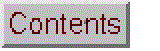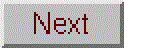# Animated Diffraction grating

### Microsoft Excel and OpenOffice Calc Versions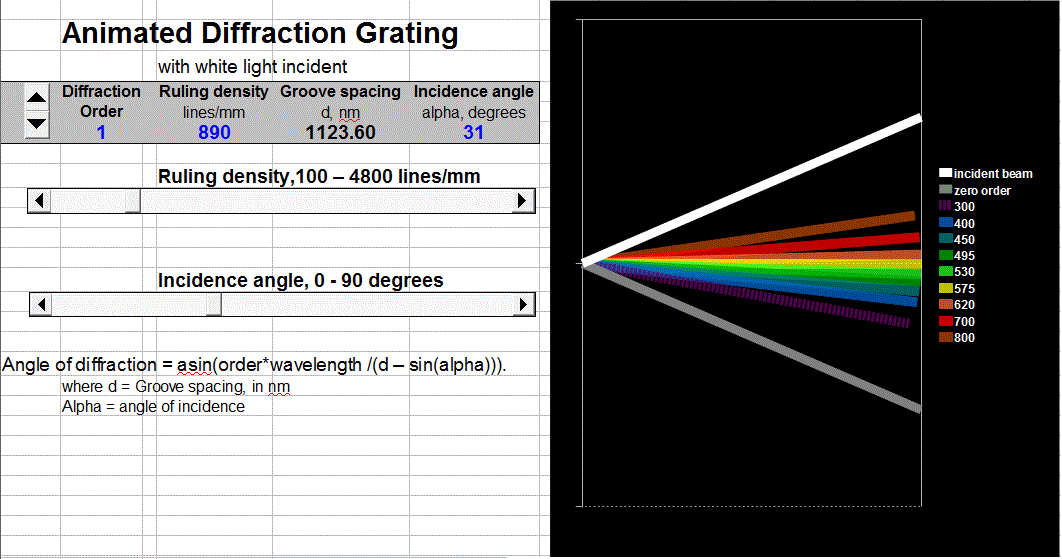Fixed grating. Download Excel or Calc version.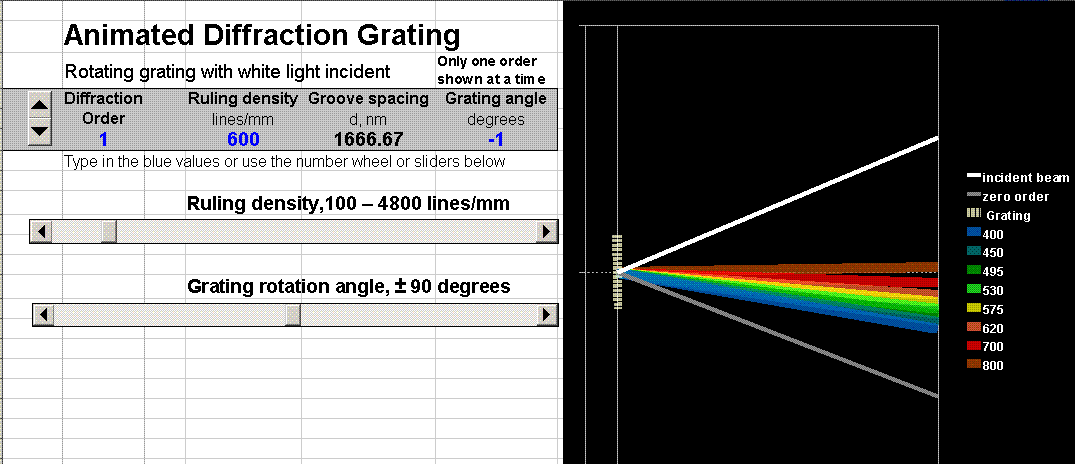Rotating grating. Download Excel or Calc version.

Animated model of a plane diffraction grating, with sliders to control the angle of incidence, the ruling density, and the diffraction order viewed. Based on the grating equation: Angle of diffraction = asin(order*wavelength /(groove spacing) - sin(angle of incidence)).

Assumptions: The grating surface is represented by the striped bar on the left of the figure. It is illuminated with a thin parallel, collimated "white" light beam consisting of eight discrete wavelengths. Only one diffraction order is shown at a time (selected by the number wheel). Only eight wavelengths are shown in the diffracted beam. The colors of the diffracted beams roughly match the wavelengths of those beams. The relative intensity of different wavelengths and orders is not represented.  For clarity, narrow beams are shown, even though in practical applications a wide beam, entirely covering the grating surface, is ordinarily used. Angles are measured from the horizontal axis.

View Equations (.pdf)

Fixed grating with variable angle of incidence

Rotating grating (as in Ebert or Czerny-Turner mounting), shown above.

There is also an alternative version with 4 different pairs of wavelengths separated by 1 nm, useful for demonstrating the conditions leading to the highest dispersion.  The angular dispersion of a grating is determined by differentiating the diffraction angle with respect to wavelength and is equal to order*(ruling density) /cos(angle of diffraction), where the ruling density is the number of grating lines per unit length.  As the angle of diffraction approaches 90º, its cosine approaches zero, and the angular dispersion approaches infinity.  So the dispersion is expected to be greatest for whatever wavelength is diffracted close to 90º. Of course, you can't actually use a diffraction angle of 90 degrees, but you should be able to get close.  If you achieve a sufficiently high angular resolution, you'll be able to separate the diffracted beams into two close-spaced wavelengths. Try it!

### Wingz Version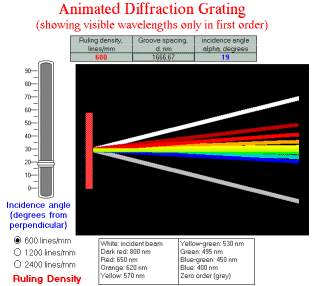WingZ version Grat1.wkz
[OpenOffice and Excel Versions]

Four "Animated figure" models that help students understand diffraction gratings in stationary and Czerny-Turner mountings. There are four variations of this model:

• Grat1 is a stationary grating with incident white light, pictured above. Eight colors of diffracted light shown in the first order only, plus the zeroth order reflection shown as a gray line. The colors of the diffracted beams roughly match the wavelengths of those beams. Students can change the angle of the incident beam and the grating ruling density. Download links: Grat1.WKZ. This model is also available in OpenOffice format (.ods) and in Excel format (.xls).
• Grat2 is a stationary grating with incident white light, used to demonstrate the relationship between the first and second orders of diffraction. Six visible colors of diffracted light shown in the first and second orders, plus the zeroth order reflection shown as a gray line. The colors of the diffracted beams roughly match the wavelengths of those beams. Students can change the angle of the incident beam and the grating ruling density. Download link: grat2.wkz
• Grating is a stationary grating with monochromatic incident light. Diffraction in the zeroth, first, and second orders are shown. The diffracted beams are shown in shades or gray and patterns; no use of color is made. Students can change the angle of incidence, the wavelength of the incident light beam, and the grating ruling density. Download link: Grating.wkz.
• CzernyTurner is a rotating grating in a Czerny-Turner mounting. Diffraction in the zeroth, first, and second orders are shown. The diffracted beams are shown in shades or gray and patterns; no use of color is made. Students can change the angle of rotation of the grating, the angle between the incident beam and observed diffracted beans, the wavelength of the incident light beam, and the grating ruling density. Download link: CzernyTurner.wkz.
These models can be operated using only the mouse-activitated on-screen sliders and radio buttons, useful when used in a lecture-demonstration environment with a computer video projection system, in a darkened room, where it is difficult to use the keyboard data entry .

Wingz player application and basic set of simulation modules, for windows PCs or Macintosh

There is also a set of diffraction grating models written in Matlab. See below for Excel and OpenOffice Calc versions.

### Mathematical basis

Inputs:
(All angles measured from the perpendicular to the grating surface.)
Angle of incidence = alpha, degrees, changed by typing into entry table or by slider
Ruling density = R, lines/mm, changed by typing into entry table or by radio buttons

Calculated quantities:
Groove spacing = d, 1000000/R (displayed in nm in table).
alphar = alpha/(360/(2*pi()))
Angle of diffraction = asin(order*wavelength/d-sin(alphar))

(c) 1991, 2015. This page is part of Interactive Computer Models for Analytical Chemistry Instruction, created and maintained by Prof. Tom O'Haver , Professor Emeritus, The University of Maryland at College Park. Comments, suggestions and questions should be directed to Prof. O'Haver at toh@umd.edu. Number of unique visits since May 17, 2008: